# McDougal Littell Geometry Chapter 5: Properties of Triangles Chapter Exam

Exam Instructions:

Choose your answers to the questions and click 'Next' to see the next set of questions. You can skip questions if you would like and come back to them later with the yellow "Go To First Skipped Question" button. When you have completed the practice exam, a green submit button will appear. Click it to see your results. Good luck!

### Page 1

#### Question 1 1. Which statement is true regarding areas based on the pictured triangle? Point G is the centroid.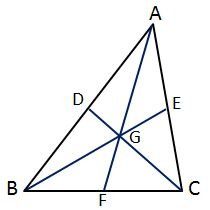#### Question 2 2. In the pictured triangle, what is a median?#### Question 3 3. What type of line segment is represented by the dotted line in the pictured triangle?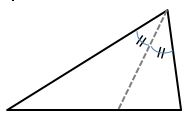#### Question 4 4. In the pictured triangle, FE is an angle bisector. What is the length of GE?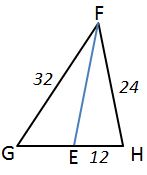#### Question 5 5. Find the measure of MN: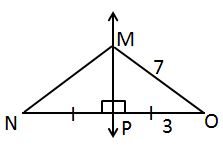### Page 2

#### Question 6 6. In the pictured triangle, XV is an angle bisector. What is the length of XY?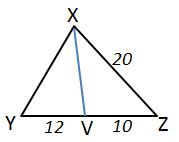#### Question 7 7. Which statement is true regarding areas based on the pictured triangle? Point G is the centroid.#### Question 9 9. In the pictured triangle, AD is an angle bisector. What is the length of AC?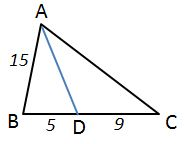#### Question 10 10. In the pictured triangle, MS is an angle bisector. What is the length of SO?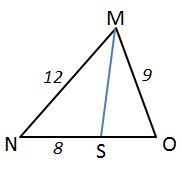### Page 3

#### Question 11 11. What type of line segment is represented by the dotted line in the pictured triangle?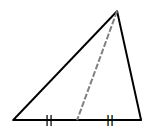#### Question 12 12. Which statement is true about the pictured triangle?#### Question 13 13. AD is a perpendicular bisector. Find the measure of AC: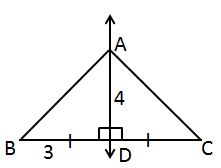#### Question 14 14. Find the measure of XZ: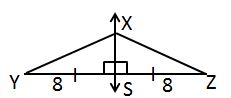#### Question 15 15. In the pictured triangle, which of the following lines is an altitude?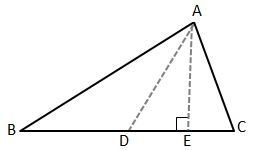### Page 4

#### Question 16 16. What type of line segment is represented by the vertical dotted line in the pictured triangle?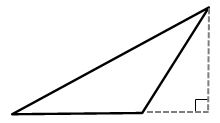#### Question 17 17. In the pictured triangle, identify the altitude(s).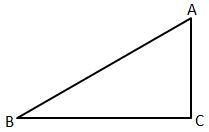#### Question 18 18. Which of the following represents the angle bisector theorem for the pictured triangle?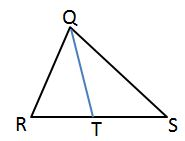#### Question 19 19. In the pictured triangle, what is the centroid?#### McDougal Littell Geometry Chapter 5: Properties of Triangles Chapter Exam Instructions

Choose your answers to the questions and click 'Next' to see the next set of questions. You can skip questions if you would like and come back to them later with the yellow "Go To First Skipped Question" button. When you have completed the practice exam, a green submit button will appear. Click it to see your results. Good luck!

Support